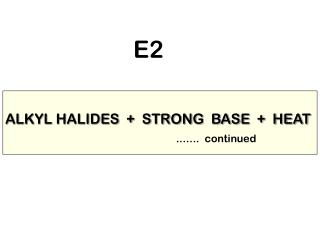DownloadDownload PresentationALKYL HALIDES + STRONG BASE + HEAT

ALKYL HALIDES + STRONG BASE + HEAT

Télécharger la présentationALKYL HALIDES + STRONG BASE + HEAT

- - - - - - - - - - - - - - - - - - - - - - - - - - - E N D - - - - - - - - - - - - - - - - - - - - - - - - - - -
Presentation Transcript

1. E2 ALKYL HALIDES + STRONG BASE + HEAT ....... continued

2. SUMMARY TO DATE ELIMINATION REACTIONS OF ALKYL HALIDES STRONG BASE Required HEAT Usually required REGIOSELECTIVE Follows Zaitsev Rule (Unless Stereochemistry Prevents) - favors most substituted alkene STEREOSPECIFIC b-H and X must be ANTI-COPLANAR - acyclics may have to rotate - rings may have to invert

3. KINETICS Examination of the rate expression often helps to understand the mechanism. The rate expression is determined by experiment.

4. RATE EXPRESSIONS exponents n m K [A] [B] RATE = concentrations of reactants A and B in moles / liter rate “constant” Actually will change with temperature and solvent, the specific molecule, etc. REACTION ORDER = SUM OF EXPONENTS = n + m Not all reactants will necessarily show up in the rate expression. 3/2 2 Fractional rate orders are possible : RATE = K [A] [B]

5. CH3CH2 CH CHCH3 CH3CH2 CH CHCH3) This equation explains the rate behavior : Rate = K [RBr] [OCH3] KINETICS CH3CH2CH2 CH + NaOCH3 CH3 35oC Br + CH3OH Rate = + d ( dt REACTION RATE DATA Rate [RBr] [OCH3] x1 x1 x1 x2 x2 x1 x2 x1 x2 x4 x2 x2 x8 x4 x2 x9 x3 x3 second order rate

6. MECHANISM Elimination Bimolecular strong base E 2 alkyl halide CONCERTED = only one step All bonds are broken and formed without the formation of any intermediates.

7. Reaction Order Sum of the exponents of the concentration terms in the rate expression. Molecularity Number of species that come together in the rate-determining step. Rate-determining Step The slowest step in the reaction sequence. Transition State An energy highpoint in the energy profile of a reaction. Activated Complex The species that exists at the transition state.

8. E2 CONCERTED REACTION One Step - No Intermediates

9. E2 ELIMINATION d- d- activated complex mechanism Concerted : everything happens at once with- out any intermediates.

10. Concerted (one step) reaction transition state TS E N E R G Y activation energy Ea heat of reaction DH starting material product This is what E2 looks like.

11. ORBITAL ALIGNMENT IN MOST CONCERTED REACTIONS THE ORBITALS BECOME PREALIGNED FOR A SMOOTH PROGRESSION OF EVENTS The anti-coplanar arrangement of theb-H and the halide leaving group X places the orbitals that undergo change in a perfect alignment. The coplanar arrangement allows a continuous movement of electrons from one end of the system to the other, much like a stack of dominoes each pushing the next one over. The two orbitals that will form the pi bond are already parallel (anti-coplanar) so that the double can form easily.

12. .. When these electrons enter the back lobe of the adjacent orbital they “push” the bonding pair out the other end (along with Br). CH3 : O .. H .. R The critical event is the removal of the b-H. sp3 H C C H R .. sp3 : : Br .. The attack of the base on the b-hydrogen starts the reaction. Notice the parallel aligment of the two sp3 orbitals.

13. Note the parallel orbitals in the pi bond. .. CH3 O H .. . . 2p 2p H R C C R H .. : : Br The formation of the double bond and the loss of bromide finish it. ..

14. FRONTIER MO THEORY The LUMO is present on the b-H only in the anti -coplanar arrangement.

15. ANTI CONFORMATION LUMO has density on H RECALL : - B: Frontier theory requires a base or nucleophile to add to the LUMO. LUMO - B: HOMO LUMO negative end of molecule DENSITY-ELPOT

16. SYN CONFORMATION - B: LUMO has no density on any H DENSITY-ELPOT LUMO

17. KINETIC ISOTOPE EFFECT The reaction slows if the b-H is replaced by D. This “kinetic isotope effect” shows that breaking the b C-H bond is a part of the rate-determining step.

18. ISOTOPES OF HYDROGEN NAME SYMBOL MASS COMPOSITION H 1 1 proton + 1 electron PROTIUM 99.985% D DEUTERIUM 2 1 proton + 1 neutron + 1 electron 0.015% T 1 proton + 2 neutrons + 1 electron TRITIUM 3 radioactive ( b- ) 12.26 yrs

19. ISOTOPE EFFECT B B H D C-H C-D H C H C H C H C B r B r C-D bond is stronger than C-H kH Slows the reaction if breaking this bond is part of the rate-determining step. approx. 5-8 kD for an isotope effect

20. ORIGIN OF THE ISOTOPE EFFECT The effect is due to differences in C-H and C-D bond strengths.

21. + + vibrational energy levels + + zero-point energy CH CD + + average bond length BONDING CURVE (D) 1s C(sp3) (H)1s + + r ENERGY + = nucleus Since D is heavier than H the C-D bond vibrates slower over a shorter distance. o o r (bond distance)

22. C-D BONDS ARE STRONGER THAN C-H BONDS bond breaks here ENERGY bond dissociation energies (CD > CH) CH CD o o r (bond distance) D is heavier than H and the CD bond vibrates more slowly over a shorter distance than CH.

23. OTHER ELIMINATION MECHANISMS

24. Three types of elimination reactions are conceivable concerted E2 just studied carbocation E1 halogen first proton second carbanion E1cb proton first halogen second